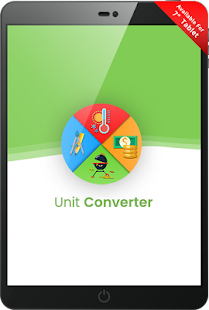Simple and easy to use currency and Easy unit converter app to handle any conversion you need.

Easy Unit Converter covers : Currency, Unit Lookup, Acceleration, Angle, Area, Data Storage, Data Transfer Speed, Density, Energy, Force, Frequency, length/Distance, Mass/Weight, Power, Prefix, Pressure, Speed/Velocity, Temperature, Time, Torque, Volume and many more.

Easy Unit Converter: Below is the list of supported conversion categories, all convert metric to imperial units and imperial to metric transformations:
✓ Energy: power, temperature conversions (Celsius, Fahrenheit), consumed energy.
✓ Electricity: electric charge, electric current, electric capacitance, electric potential, electric resistance.
✓ Basic dimension: length (distance), area, volume.
✓ For daily use: currency converter, clothes and shoe sizes for men, women and children, cooking weights, fuel consumption and time.
✓ Mechanics: pressure, weight, force, torque.
✓ Motion: speed, acceleration, angular speed, flow rate
✓ Chemistry: density, dynamic and kinematic viscosity, mineralization.
✓ Computing: data storage and data transfer.

Easy Unit Converter: The Complete List of Measurement Units Supported :
– Mass and Weight
– Dollar Rate Calculator
– Foreign Currency Converter
– Exchange Rate Calculator
– Distance and Length
– Capacity and Volume
– Area Unit Converter
– Speed Unit Converter
– Temperature Conversion
– Simple Unit Conversion
– Currency Converter
– Length & Weight Unit Converter
– Measurement Converter
– Conversion Calculator
– Metric Conversion Calculator
– Area Converter
– Metric Measurements
– Pressure, Volume Conversion
– Metric System Conversion
– Metric Calculator
– Conversion of Measurement Units
– Converting Metric Units
– Imperial to Metric Conversion
– Weight Conversion
– Measurement Conversion
– Metric to Standard Conversion
– Convert Cm To In – In To Cm
– Conversion of Measurement Units
– Length Conversion
– Measurement Conversion Calculator
– Pressure Unit Conversion
– Convert Inch to Cm
– Standard to Metric Conversion
– Measurement Calculator
– Weight Conversion Calculator
– Convert Lbs to Kg – Kg to Lbs
– Engineering Unit Converter
– Torque Unit Converter
– Inch Unit Converter
– Units of Measurement
– Convert – Exchange Rate Calculator
– Currency Exchange
– Money Converter, Exchange
– Foreign Exchange Converter
– Currency Rate Conversion

Feedbacks and suggestions
If you enjoy using Easy Unit Converter App, would you mind taking a moment to rate it? It won’t take more than a minute. Thanks for your support!

What’s New
Easy Unit Converter covers : Currency, Unit Lookup, Acceleration, Angle, Area, Data Storage, Data Transfer Speed, Density, Energy, Force, Frequency, length/Distance, Mass/Weight, Power, Prefix, Pressure, Speed/Velocity, Temperature, Time, Torque, Volume and many more.

Screenshots

•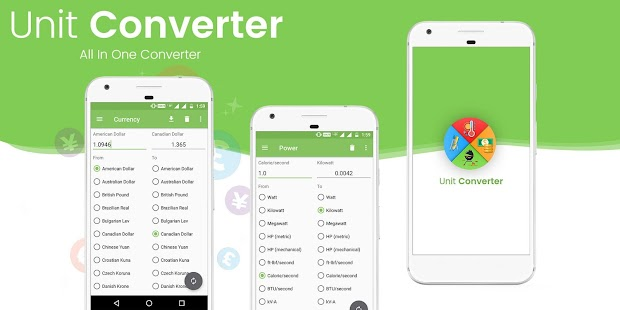•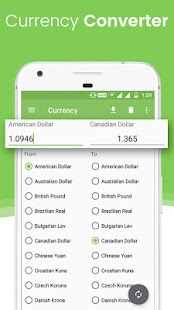•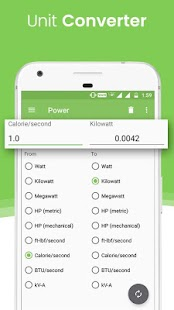•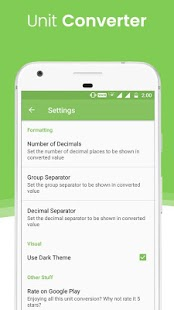•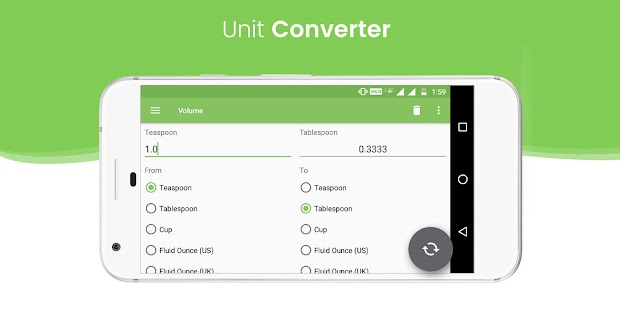•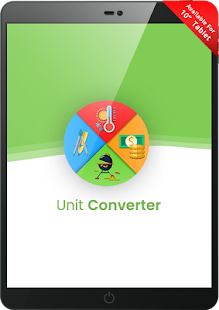•